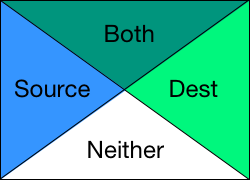Ever since I dabbled around css blend modes, I wanted to look into the math behind it.

## Compositing in CSS

Compositing is combining separate visual elements into a single image. There have been 2 types of compositing techniques used in CSS so far.

Simple Alpha Compositing Porter-Duff Compositing
Mixing effect is obtained by overlapping of transparent layers Colors are mixed by blending functions and resultant region is obtained by Porter-Duff source-over operator
Used by old versions of CSS Used in CSS3*

## Porter-Duff Compositing

When 2 pixels get combined, 4 subpixel regions are formed:

• Region where only source is present
• only destination is present
• both are present
• neither is present (always empty)There are 12 of operators which decides the behaviour in these regions.

Porter-Duff Operations (source S on backdrop B)
No. Operation Quadraple Diagram FS FB
1 Clear (0,0,0,0)0 0
2 S (0,S,0,S)1 0
3 B (0,0,B,B)1 0
4 S over B (0,S,B,S)1 1 - αS
5 B over S (0,S,B,B)1 - αB 1
6 S in B (0,0,0,S)αB 0
7 B in S (0,0,0,B)0 αS
8 S out B (0,S,0,0)1 - αB 0
9 B out S (0,0,B,0)0 1 - αS
10 S atop B (0,0,B,S)αB 1 - αA
11 B atop S (0,S,0,B)1 - αB αS
12 S xor B (0,S,B,0)1 - αB 1 - αS
CSS is using the Souce over Backdrop operation (4th entry in the table above) for blend modes.

Color obtained from compositing source color Cs and backdrop color Cb is given by general Porter Duff equation:

``````co = αs x Fs x Cs + αb x Fb x Cb
αo = αs x 1 + αb x (1 – αs)
``````

## Blending

Blending is the aspect of compositing that calculates the mixing of colors where the source element and backdrop overlap.

``````Cr = (1 - αb) x Cs + αb x B(Cb, Cs)
``````

where B(Cb, Cs) is the blending function.

Blend mode Calculation
normal
`B(Cb, Cs) = Cs`
multiply
`B(Cb, Cs) = Cb x Cs`
screen
`B(Cb, Cs) = 1 - [(1 - Cb) x (1 - Cs)]= Cb + Cs -(Cb x Cs)`
overlay
`B(Cb, Cs) = HardLight(Cs, Cb)`
darken
`B(Cb, Cs) = min(Cb, Cs)`
lighten
`B(Cb, Cs) = max(Cb, Cs)`
color-dodge
```if(Cb == 0)
B(Cb, Cs) = 0
else if(Cs == 1)
B(Cb, Cs) = 1
else
B(Cb, Cs) = min(1, Cb / (1 - Cs))```
color-burn
```if(Cb == 1)
B(Cb, Cs) = 1
else if(Cs == 0)
B(Cb, Cs) = 0
else
B(Cb, Cs) = 1 - min(1, (1 - Cb) / Cs)
```
hard-light
```if(Cs <= 0.5)
B(Cb, Cs) = Multiply(Cb, 2 x Cs)
else
B(Cb, Cs) = Screen(Cb, 2 x Cs -1)
```
soft-light
```if(Cs <= 0.5)
B(Cb, Cs) = Cb - (1 - 2 x Cs) x Cb x (1 - Cb)
else
B(Cb, Cs) = Cb + (2 x Cs - 1) x (D(Cb) - Cb)
with
if(Cb <= 0.25)
D(Cb) = ((16 * Cb - 12) x Cb + 4) x Cb
else
D(Cb) = sqrt(Cb)
```
difference
`B(Cb, Cs) = | Cb - Cs |`
exclusion
`B(Cb, Cs) = Cb + Cs - 2 x Cb x Cs`
hue
`h(Cs), s(Cb), l(Cb)`
saturation
`h(Cb), s(Cs), l(Cb)`
color
`h(Cs), s(Cs), l(Cb)`
luminosity
`h(Cb), s(Cb), l(Cs)`

Here is a demo for blend mode generator in javascript:

See the Pen CSS Blend Modes: Generator in JS by Praseetha KR (@Praseetha-KR) on CodePen.